# 1 Overview

This vignette walks through 3 analysis examples using data accessed with the `recountmethylation` package. First, predicted and chronological ages are compared from the sample metadata. Then quality signals (methylated and unmethylated, log2 median scale) are compared between samples stored using either formalin fixed paraffin-embedding (FFPE) or freezing. Finally, tissue-specific probe sets with high DNA methylation (DNAm) fraction variances are identifed and analyzed using liver and adipose samples. Note that versions of these analyses also appear in the manuscript Maden et al. (2020).

## 1.1 Analysis script and limited chunk evaluation

This vignette accompanies the “data_analyses.R” script. Note the script was written with extensibility to new and larger comparator groups in mind. While the script should run to completion without errors, it takes several hours in total to complete (excluding the time to download large database files). Due to this lengthy script run time, this vignette only evaluates code chunks utilizing final/resultant data objects produced by the script (e.g. for tables, tests, and figures). For completeness, remaining script steps and code are included but not evaluated.

Load the file “data_analyses.RData” from the `recountmethylation` package files. This contains the resultant/final data objects produced by the script, which will be used in evaluated code chunks below.

``````sf <- system.file(file.path("extdata", "data_analyses"),
package = "recountmethylation")
load(file.path(sf, "data_analyses.RData"))``````

## 1.2 Datasets and data objects

The analysis script uses sample metadata and 2 database files. Retrieve the provided sample metadata from the `recountmethylation` package files.

``````# get local metadata
mdpath <- system.file("extdata", "gsm_metadata", "md_final_hm450k_0-0-1.rda",
package = "recountmethylation")
md <- get(load(mdpath))``````

Also obtain 2 `HDF5-SummarizedExperiment` database files, the GenomicRanges and MethylSet files. Consult the `users_guide` vignette for details about the database file formats and download instructions. Once the datasets downloaded, they can be loaded into an R session as follows.

``````# load methylset
gmdn <- "remethdb-h5se_gm_0-0-1_1590090412"
gm <- loadHDF5SummarizedExperiment(gmdn)
# load grset
grdn <- "remethdb-h5se_gr_0-0-1_1590090412"
gr <- loadHDF5SummarizedExperiment(grdn)``````

# 2 Example 1: Comparing mined and predicted age

This example uses sample metadata to compare mined and predicted ages from the `age` and `predage` variables, respectively. Values in `age` were mined from GEO record metadata and are included with available age units. Values in `predage` were calculated from noob-normalized (Triche et al. (2013)) DNAm Beta-values with `agep`, a function from the `wateRmelon` package that implements the Horvath biological age clock (Horvath (2013)).

## 2.1 Make new variables and filter samples

Get samples for which both `age` and `predage` age are available. From `age`, make a new numeric variable `chron.age`.

``````mdf <- md[!md\$age == "valm:NA",]
mdf\$chron.age <- as.numeric(gsub(";.*", "", gsub("^valm:", "", mdf\$age)))
mdf\$predage <- as.numeric(mdf\$predage)
mdf <- mdf[!is.na(mdf\$chron.age),]
mdf <- mdf[!is.na(mdf\$predage),]``````

Next, make a new variable `stype` from `sampletype` and remove samples with missing values.

``````mdf\$stype <- as.character(gsub(";.*", "",
gsub("^msraptype:", "", mdf\$sampletype)))
mdf <- mdf[!is.na(mdf\$stype),]``````

Now make a new variable `is.cx` from querying `cancer` in the `disease` term. This reflects whether a sample was likely from a cancer or a cancer patient.

``mdf\$is.cx <- ifelse(grepl(".*cancer.*", mdf\$disease), TRUE, FALSE)``

Next, store the study-wise age differences in the `xdif` variable using the mean absolute difference (a.k.a. “MAD”) between `chron.age` and `predage` across samples from the same study. Also store study sizes in the `ngsm` term for plotting.

``````xdif <- ngsm <- c()
for(g in unique(mdf\$gseid)){
mdff <- mdf[mdf\$gseid==g, ]
xdif <- c(xdif, mean(abs(mdff\$chron.age - as.numeric(mdff\$predage))))
ngsm <- c(ngsm, nrow(mdff))
}
names(xdif) <- names(ngsm) <- unique(mdf\$gseid)``````

Make a new filtered `mdff` data frame using the new variables. Retain likely non-cancer samples from studies with MAD <= 10 years. Pre- and post-filter datasets (groups 1 and 2, respectively) are summarized below.

``````filt <- mdf\$stype == "tissue" & !mdf\$is.cx
filt <- filt & !mdf\$gseid %in% names(xdif[xdif > 10])
mdff <- mdf[filt, ]``````

## 2.2 Analyses and summary statistics

Perform statistical analyses of `mdf` (group 1) and `mdff` (group 2). First, generate multiple regressions for each.

``````lm1 <- lm(mdf\$predage ~ mdf\$chron.age + mdf\$gseid + mdf\$stype + mdf\$is.cx)
lm2 <- lm(mdff\$predage ~ mdff\$chron.age + mdff\$gseid)``````

Now perform analyses of variances (ANOVAs) on multiple regressions. Summarize variance percentages and p-values for covariates in each model. Columns “Vperc” and “Pval” are the percent variance and unadjusted p-value for covariates in each model.

``````# anovas
av1 <- anova(lm1)
av2 <- anova(lm2)
# results summaries
sperc1 <- round(100*av1\$`Sum Sq`[1:4]/sum(av1\$`Sum Sq`), 2)
pval1 <- format(av1\$`Pr(>F)`[1:4], scientific = TRUE, digits = 3)
sperc2 <- round(100*av2\$`Sum Sq`[1:2]/sum(av2\$`Sum Sq`), 2)
pval2 <- format(av2\$`Pr(>F)`[1:2], scientific = TRUE, digits = 3)
# summary table
dan <- data.frame(Vperc1 = c(sperc1),
Pval1 = c(pval1),
Vperc2 = c(sperc2, "-", "-"),
Pval2 = c(pval2, "-", "-"),
stringsAsFactors = FALSE)
rownames(dan) <- c("Chron.Age", "GSEID", "SampleType", "Cancer")
knitr::kable(dan, align = "c")``````
Vperc1 Pval1 Vperc2 Pval2
Chron.Age 51.73 0.00e+00 92.77 0.00e+00
GSEID 24.40 0.00e+00 1.52 2.42e-274
SampleType 0.07 1.27e-09 - -
Cancer 0.01 1.55e-02 - -

Now calcualte the R-squared, Spearman correlation coefficient (Rho), and MAD for each model.

``````# rsquared
rsq1 <- round(summary(lm1)\$r.squared, 2)
rsq2 <- round(summary(lm2)\$r.squared, 2)
# correlation coefficient
rho1 <- round(cor.test(mdf\$predage, mdf\$chron.age,
method = "spearman")\$estimate, 2)
rho2 <- round(cor.test(mdff\$predage, mdff\$chron.age,
test = "spearman")\$estimate, 2)
# mean absolute difference
mad1 <- round(mean(abs(mdf\$chron.age - mdf\$predage)), 2)
mad2 <- round(mean(abs(mdff\$chron.age - mdff\$predage)), 2)``````

Finally, organize and display the results

``````dss <- data.frame(group = c("1", "2"),
ngsm = c(nrow(mdf), nrow(mdff)),
ngse = c(length(unique(mdf\$gseid)),
length(unique(mdff\$gseid))),
r.squared = c(rsq1, rsq2), rho = as.character(c(rho1, rho2)),
mad = c(mad1, mad2), stringsAsFactors = FALSE)
knitr::kable(dss, align = "c")``````
group ngsm ngse r.squared rho mad
1 16510 105 0.76 0.76 12.87
2 6019 37 0.94 0.96 4.53

## 2.3 Scatter plots of study errors and sample ages

Plot sample counts and MAD for each GSE record, with a vertical line at the 10-years MAD cutoff used for the group 2 filter.

``````plot(xdif, ngsm, ylab = "Study Size (Num. GSM)",
xlab = "Age Difference, MAD[Chron, Pred]")
abline(v = 10, col = "red")``````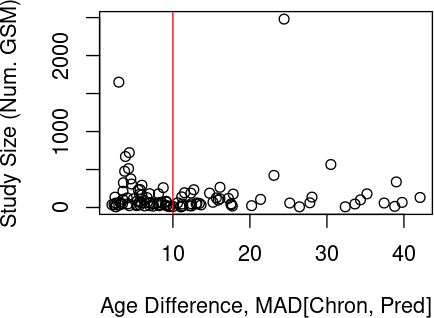Finally, plot the chronological and predicted ages for group 2 samples.

``````ggplot(mdff, aes(x = chron.age, y = predage)) +
geom_point(size = 1.2, alpha = 0.2) + geom_smooth(method = "lm", size = 1.2) +
theme_bw() + xlab("Chronological Age") + ylab("Epigenetic (DNAm) Age")``````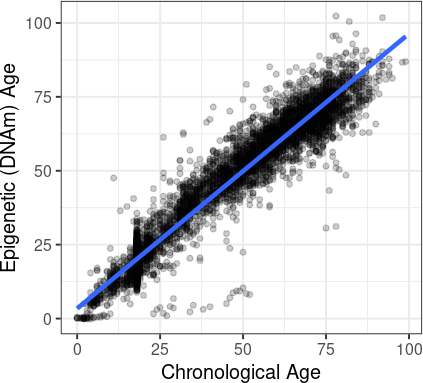# 3 Example 2: Signal comparison of FFPE and frozen samples

This section compares methylated and unmethylated signal (log2 sample median scale) between samples stored with either FFPE or fresh freezing (FF).

## 3.1 Get samples with storage type information

Identify and summarize samples with the `storage` variable available. Use values in `storage` to inform a new `sgroup` variable.

``````mdf <- md[!md\$storage == "NA",]
mdf\$sgroup <- ifelse(grepl("FFPE", mdf\$storage), "ffpe", "frozen")``````
``````# get summary table
sst <- get_sst(sgroup.labs = c("ffpe", "frozen"), mdf)
knitr::kable(sst, align = "c") # table display``````

## 3.2 Use blocking to calculate signal log2 medians

Subset the `MethylSet` object and extract the full signal matrices with the `getMeth` and `getUnmeth` functions from the minfi package.

``````gmf <- gm[, gm\$gsm %in% mdf\$gsm] # filt h5se object
mdf <- mdf[order(match(mdf\$gsm, gmf\$gsm)),]
identical(gmf\$gsm, mdf\$gsm)
gmf\$storage <- mdf\$storage # append storage info``````
``````meth.all <- getMeth(gmf)
unmeth.all <- getUnmeth(gmf)``````

Next, prepare to calculate log2 median signals. To manage data in active memory, process it in smaller units or blocks. Using the `get_blocks` helper function, assign sample indices to blocks of size 1,000 using the `bsize` argument.

``blocks <- getblocks(slength = ncol(gmf), bsize = 1000)``

Now calculate log2 of sample median signals for each block. Vectorize calculations within blocks with `apply`. Store results in the data.frame `ds`.

``````ms <- matrix(nrow = 0, ncol = 2)
l2meth <- l2unmeth <- c()
for(i in 1:length(blocks)){
b <- blocks[[i]]
gmff <- gmf[, b]
methb <- as.matrix(meth.all[, b])
unmethb <- as.matrix(unmeth.all[, b])
l2meth <- c(l2meth, apply(methb, 2, function(x){
log2(median(as.numeric(x)))
}))
l2unmeth <- c(l2unmeth, apply(unmethb, 2, function(x){
log2(median(as.numeric(x)))
}))
ms <- rbind(ms, matrix(c(l2meth, l2unmeth), ncol = 2))
message(i)
}
rownames(ms) <- colnames(meth.all)
colnames(ms) <- c("meth.l2med", "unmeth.l2med")
ds <- as.data.frame(ms)
ds\$storage <- ifelse(grepl("FFPE", gmf\$storage), "ffpe", "frozen")``````

## 3.3 Signals plotted by storage type

Evaluate signal patterns across storage type using plots using the ggplot2 package. First, make a 2d scatter plot of methylated and unmethylated signals using the `geom_point` function. Color by storage type with the `scale_color_manual` function (FFPE samples are orange, frozen samples are purple).

``````ggplot(ds, aes(x = meth.l2med, y = unmeth.l2med, color = storage)) +
geom_point(alpha = 0.35, cex = 3) + theme_bw() +
scale_color_manual(values = c("ffpe" = "orange", "frozen" = "purple"))``````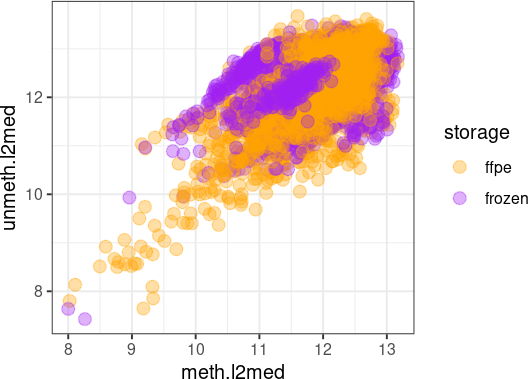Next, make separate violin plots for signals and groups using the `geom_violin` function with the same colors for each storage type. Draw horizontal median lines by setting the `draw_quantiles` argument to 0.5.

``````vp <- matrix(nrow = 0, ncol = 2)
vp <- rbind(vp, matrix(c(ds\$meth.l2med, paste0("meth.", ds\$storage)),
ncol = 2))
vp <- rbind(vp, matrix(c(ds\$unmeth.l2med, paste0("unmeth.", ds\$storage)),
ncol = 2))
vp <- as.data.frame(vp, stringsAsFactors = FALSE)
vp[,1] <- as.numeric(vp[,1])
colnames(vp) <- c("signal", "group")
vp\$col <- ifelse(grepl("ffpe", vp\$group), "orange", "purple")
# make plot
ggplot(vp, aes(x = group, y = signal, color = group)) +
scale_color_manual(values = c("meth.ffpe" = "orange",
"unmeth.ffpe" = "orange", "meth.frozen" = "purple",
"unmeth.frozen" = "purple")) +
geom_violin(draw_quantiles = c(0.5)) + theme_bw() +
theme(legend.position = "none")``````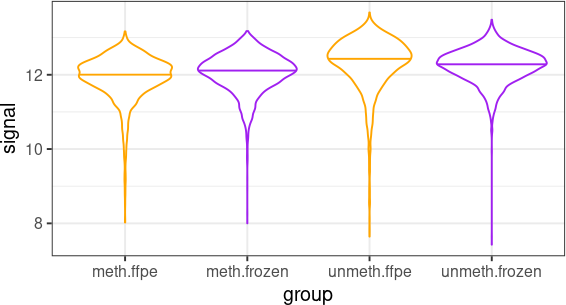# 4 Example 3: Identify and analyze tissue-specific probes with the highest

variances

This example describes variance analyses in liver and adipose, 2 of the 7 tissues analyzed in the manuscript Maden et al. (2020). This includes a quality assessment, study ID linear adjustment of DNAm fractions, ANOVA-based and probe filtering, 2-step variance analyses, and results plots.

## 4.1 Sample identification and summary

Summarize the samples of interest. Use two vectors of GSM IDs, `adipose.gsmv` and `liver.gsmv` to filter the metadata (see vectors in the data_analyses.R script). Also define tissues in the new group variable `sgroup`. Summarize the sample groups in a table

``````gsmv <- c(adipose.gsmv, liver.gsmv)
mdf <- md[md\$gsm %in% gsmv,]
mdf\$sgroup <- ifelse(mdf\$gsm %in% adipose.gsmv, "adipose", "liver")
sst.tvar <- get_sst(sgroup.labs = c("liver", "adipose"), mdf)
knitr::kable(sst.tvar, align = "c")``````
liver adipose
ngsm 112 104
meangsm.gse 16 26
sdgsm.gse 17.3 19.41
numgse 7 4
min.predage 1.91 36.07
max.predage 73.75 79.27
mean.predage 42.72 54.17
sd.predage 17.06 7.63
numna.predage 0 0
percfemale.predsex 45.54 68.27
numna.predsex 0 0

## 4.2 Calculate log2 methylated and unmethylated signal medians

Subset the `MethylSet` dataset, then append the `sgroup` variable from `mdf` and map the object to the genome using the `mapToGenome` function from the `minfi` package.

``````ms <- gm[,colnames(gm) %in% rownames(mdf)]
ms <- ms[,order(match(colnames(ms), rownames(mdf)))]
identical(colnames(ms), rownames(mdf))
#  TRUE
ms\$sgroup <- mdf\$sgroup
ms <- mapToGenome(ms)
dim(ms)
#  485512    252``````

As in example 2 above, calculate the sample log2 median signals from signal matrices. Process the data in blocks using within-block vectorization with `apply`.

``````# get log2 medians
meth.tx <- getMeth(ms)
unmeth.tx <- getUnmeth(ms)
blocks <- getblocks(slength = ncol(ms), bsize = 50)
# process data in blocks
l2m <- matrix(nrow = 0, ncol = 2)
for(i in 1:length(blocks)){
b <- blocks[[i]]
gmff <- ms[, b]
methb <- as.matrix(meth.tx[, b])
unmethb <- as.matrix(unmeth.tx[, b])
l2meth <- l2unmeth <- c()
l2meth <- c(l2meth, apply(methb, 2, function(x){
log2(median(as.numeric(x)))
}))
l2unmeth <- c(l2unmeth, apply(unmethb, 2, function(x){
log2(median(as.numeric(x)))
}))
l2m <- rbind(l2m, matrix(c(l2meth, l2unmeth), ncol = 2))
message(i)
}
ds2 <- as.data.frame(l2m)
colnames(ds2) <- c("l2med.meth", "l2med.unmeth")
ds2\$tissue <- as.factor(ms\$sgroup)``````

Make a scatter plot of log2 median signals by tissue type with the `geom_point` function.

``````ggplot(ds2, aes(x = l2med.meth, y = l2med.unmeth, color = tissue)) +
geom_point(alpha = 0.3, cex = 3) + theme_bw()``````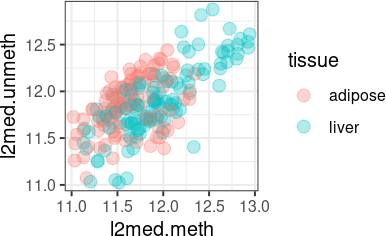## 4.3 Perform linear correction on DNAm for study IDs

Access the noob-normalized DNAm Beta-values from the `GenomicRatio` object `gr` loaded above. Extract the DNAm fractions as M-values (logit2 transformed Beta-values) with the `getM` minfi function. Perform linear correction on study ID with the `removeBatchEffect` function from the limma package by setting the `batch` argument to the “gseid” variable.

``````lmv <- lgr <- lmd <- lb <- lan <- list()
tv <- c("adipose", "liver")
# get noob norm data
gr <- gr[,colnames(gr) %in% colnames(ms)]
gr <- gr[,order(match(colnames(gr), colnames(ms)))]
identical(colnames(gr), colnames(ms))
gr\$sgroup <- ms\$sgroup
# do study ID adj
for(t in tv){
lmv[[t]] <- gr[, gr\$sgroup == t]
msi <- lmv[[t]]
madj <- limma::removeBatchEffect(getM(msi), batch = msi\$gseid)
# store adjusted data in a new se object
lgr[[t]] <- GenomicRatioSet(GenomicRanges::granges(msi), M = madj,
annotation = annotation(msi))
# append samples metadata
lmd[[t]] <- pData(lgr[[t]]) <- pData(lmv[[t]])
# append preprocessing metadata
metadata(lgr[[t]]) <- list("preprocess" = "noobbeta;removeBatchEffect_gseid")
# make betavals list
lb[[t]] <- getBeta(lgr[[t]]) # beta values list
}``````

## 4.4 Perform array-wide ANOVAs and filter probes

Prepare and run ANOVAs on autosomal probes. First, identify and remove sex chromosome probes by accessing annotation with the `getAnnotation` minfi function. List the filtered data in the `lbf` object.

``````anno <- getAnnotation(gr)
chr.xy <-c("chrY", "chrX")
cg.xy <- rownames(anno[anno\$chr %in% chr.xy,])
lbf <- list()
for(t in tv){
bval <- lb[[t]]
lbf[[t]] <- bval[!rownames(bval) %in% cg.xy,]
}
bv <- lbf[]``````

Next, select and format the 9 model covariates for the ANOVA tests. From sample metadata, select the variables for study ID (“gseid”), predicted sex (“predsex”), predicted age (“predage”), and predicted fractions of 6 cell types ("predcell..*"). Convert these to either factor or numeric type with the functions `as.factor` and `as.numeric`, respectively.

``````lvar <- list()
cnf <- c("gseid", "predsex", "predage", "predcell.CD8T",
"predcell.CD4T", "predcell.NK", "predcell.Bcell",
"predcell.Mono", "predcell.Gran")
for(t in tv){
for(c in cnf){
if(c %in% c("gseid", "predsex")){
lvar[[t]][[c]] <- as.factor(pData(lgr[[t]])[,c])
} else{
lvar[[t]][[c]] <- as.numeric(pData(lgr[[t]])[,c])
}
}
}``````

Run ANOVAs on probe Beta-values. Use the blocking-with-vectorization strategy here as above, with large blocks of 100,000 sample indices each. Calculations should complete in about 1 hour. For each test, retain unadjusted p-values and variance percentages of the 9 covariates. Store the 18-column results matrices in the `lan` list object.

``````bv <- lbf[]
blocks <- getblocks(slength = nrow(bv), bsize = 100000)
mr <- matrix(nrow = 0, ncol = 18)
lan <- list("adipose" = mr, "liver" = mr)
t1 <- Sys.time()
for(bi in 1:length(blocks)){
for(t in tv){
datr <- lbf[[t]][blocks[[bi]],]
tvar <- lvar[[t]]
newchunk <- t(apply(datr, 1, function(x){
# do multiple regression and anova
x <- as.numeric(x)
ld <- lm(x ~ tvar[] + tvar[] + tvar[] + tvar[] +
tvar[] + tvar[] + tvar[] + tvar[] + tvar[])
an <- anova(ld)
# get results
ap <- an[c(1:9),5] # pval
av <- round(100*an[c(1:9),2]/sum(an[,2]), 3) # percent var
return(as.numeric(c(ap, av)))
}))
# append new results
lan[[t]] <- rbind(lan[[t]], newchunk)
}
message(bi, "tdif: ", Sys.time() - t1)
}
# append colnames
for(t in tv){colnames(lan[[t]]) <- rep(cnf, 2)}``````

Next, remove probes showing evidence of residual confounding from the covariates. Adjust covariate p-values with the `p.adjust` function, and retain probes with adjusted p-values >= 0.001 and variance < 10% variance for all 9 covariates. Retain the filtered probe DNAm data as `GenomicRatioSet`s for each tissue in the list `lgr.filt`.

``````pfilt <- 1e-3
varfilt <- 10
lcgkeep <- list() # list of filtered probe sets
for(t in tv){
pm <- lan[[t]][,c(1:9)]
vm <- lan[[t]][,c(10:18)]
# parse variable thresholds
cm <- as.data.frame(matrix(nrow = nrow(pm), ncol = ncol(pm)))
for(c in 1:ncol(pm)){
pc <- pm[,c];
pc.adj <- as.numeric(p.adjust(pc))
pc.filt <- pc.adj < pfilt
vc.filt <- vm[,c] >= varfilt
cm[,c] <- (pc.filt & vc.filt)
}
cgkeep <- apply(cm, 1, function(x){return((length(x[x == TRUE]) == 0))})
lcgkeep[[t]] <- rownames(pm)[cgkeep]
}
lgr.filt <- list("adipose" = lgr[][lcgkeep[],],
"liver" = lgr[][lcgkeep[],])``````

## 4.5 Get probe DNAm summary statistics and analyze variances

Calculate probe DNAm summary statistics. For each tissue, calculate the minima, maxima, means, medians, standard deviations, and variances of Beta-values across samples. Store results in the `lcg.ss` list.

``````cnv <- c("min", "max", "mean", "median", "sd", "var")
bv <- getBeta(lgr.filt[[t]])
lbt <- lcg.ss <- list()
bsize = 100000
for(t in tv){
lcg.ss[[t]] <- matrix(nrow = 0, ncol = 6)
lbt[[t]] <- bt <- as.matrix(getBeta(lgr.filt[[t]]))
blockst <- getblocks(slength = nrow(bt), bsize = bsize)
for(bi in 1:length(blockst)){
bc <- bt[blockst[[bi]],]
newchunk <- t(apply(bc, 1, function(x){
newrow <- c(min(x), max(x), mean(x), median(x), sd(x), var(x))
return(as.numeric(newrow))
}))
lcg.ss[[t]] <- rbind(lcg.ss[[t]], newchunk)
message(t, ";", bi)
}
colnames(lcg.ss[[t]]) <- cnv
}``````

Perform the main variance analyses with 2 strategies. This selects the 2,000 probes with the highest group-specific variances.

First, use a single variance cutoff, or “absolute” quantile cutoff, for each group. List probes in the top 99th quantile variances for each tissue in the `lmvp.abs` object.

``````qiv = seq(0, 1, 0.01)
qwhich = c(100)
lmvp.abs <- list()
lci <- list()
for(t in tv){
cgv <- c()
sa <- lcg.ss[[t]]
sa <- as.data.frame(sa, stringsAsFactors = FALSE)
q <- quantile(sa\$var, qiv)[qwhich]
lmvp.abs[[t]] <- rownames(sa[sa\$var > q,])
}``````

Now select high-variance probes with binning for each tissue. Assign probes to 1 of 10 bins using 0.1 mean Beta-value intervals. Select probes in the top 99th variance quantiles for each bin, and store in `lmvp.bin`.

``````# binned quantiles method
qiv = seq(0, 1, 0.01) # quantile filter
qwhich = c(100)
bin.xint <- 0.1
binv = seq(0, 1, bin.xint)[1:10] # binned bval mean
# iter on ncts
lmvp.bin = list()
for(t in tv){
sa <- as.data.frame(lcg.ss[[t]])
cgv <- c()
# iterate on betaval bins
for(b in binv){
bf <- sa[sa\$mean >= b & sa\$mean < b + bin.xint, ] # get probes in bin
q <- qf <- quantile(bf\$var, qiv)[qwhich] # do bin filter
cgv <- c(cgv, rownames(bf)[bf\$var > q]) # append probes list
}
lmvp.bin[[t]] <- cgv
}``````

With the variance analyses complete, filter the `lmvp.abs` and `lmvp.bin` probes by tissue specificity. Tissue-specific probes should only occur among high variance probes for a single tissue. Categorize probes as “tissue-specific” or “non-specific” using the `table` function to determine their frequency of occurrence across tissues.

``````cgav <- c()
for(t in tv){
txcg <- unique(c(lmvp.abs[[t]], lmvp.bin[[t]]))
cgav <- c(cgav, txcg)
}
cgdf <- as.data.frame(table(cgav))
cgdf\$type <- ifelse(cgdf[,2] > 1, "non-specific", "tissue-specific")
table(cgdf\$type)``````
``````##
##    non-specific tissue-specific
##            3217            4572``````

After filtering probes by tissue specificity, rank them by descending DNAm variance. Select 1,000 probes from `lmvp.abs`, then 1,000 non-overlapping probes from `lmvp.bin`, retain the 2,000 highest-variance probes by tissue in the `ltxcg` list.

``````cgfilt <- cgdf\$type == "non-specific"
cgdff <- cgdf[!cgfilt,]
ltxcg <- list()
for(t in tv){
cgtx <- c()
cgabs <- lmvp.abs[[t]]
cgbin <- lmvp.bin[[t]]
st <- as.data.frame(lcg.ss[[t]])
# get t tissue specific probes
filtbt <- rownames(st) %in% cgdff[,1]
st <- st[filtbt,]
# get top 1k t tissue specific abs probes
filt.bf1 <- rownames(st) %in% cgabs
sf1 <- st[filt.bf1,]
sf1 <- sf1[rev(order(sf1\$var)),]
cgtx <- rownames(sf1)[1:1000]
# get top 1k t tissue specific bin probes, after filt
filt.bf2 <- rownames(st) %in% cgbin &
!rownames(st) %in% rownames(sf1)
sf2 <- st[filt.bf2,]
sf2 <- sf2[rev(order(sf2\$var)),]
cgtx <- c(cgtx, rownames(sf2)[1:1000])
ltxcg[[t]] <- cgtx
}``````

## 4.6 Violin plots and heatmaps of probe set DNAm means and variances

First, get probe set DNAm summaries and annotation data.

``````# filtered cg summaries
lfcg <- lapply(lcg.ss,
function(x){x <- x[rownames(x) %in% unique(unlist(ltxcg)),]})
# annotation subset
anno <- getAnnotation(gr) # save anno for cga
anno <- anno[,c("Name", "UCSC_RefGene_Name", "UCSC_RefGene_Group",
"Relation_to_Island")]
anno <- anno[rownames(anno) %in% unique(unlist(ltxcg)),]
# filtered beta values
lcgssf <- list()
for(t in tv){
bv <- lcg.ss[[t]]
bvf <- bv[rownames(bv) %in% ltxcg[[t]],]
lcgssf[[t]] <- bvf
}``````

Use the `makevp()` helper function to make violin plots with horizontal bars at distribution medians. This function formats the data and calls `geom_violin` to make violin plots for DNAm fraction means and variances. Store plots in the `lvp` list, then display them vertically using the `grid.arrange` function from the gridExtra package.

``````lvp <- makevp(lfcg, ltxcg)
grid.arrange(lvp[], lvp[], ncol = 1, bottom = "Tissue")``````